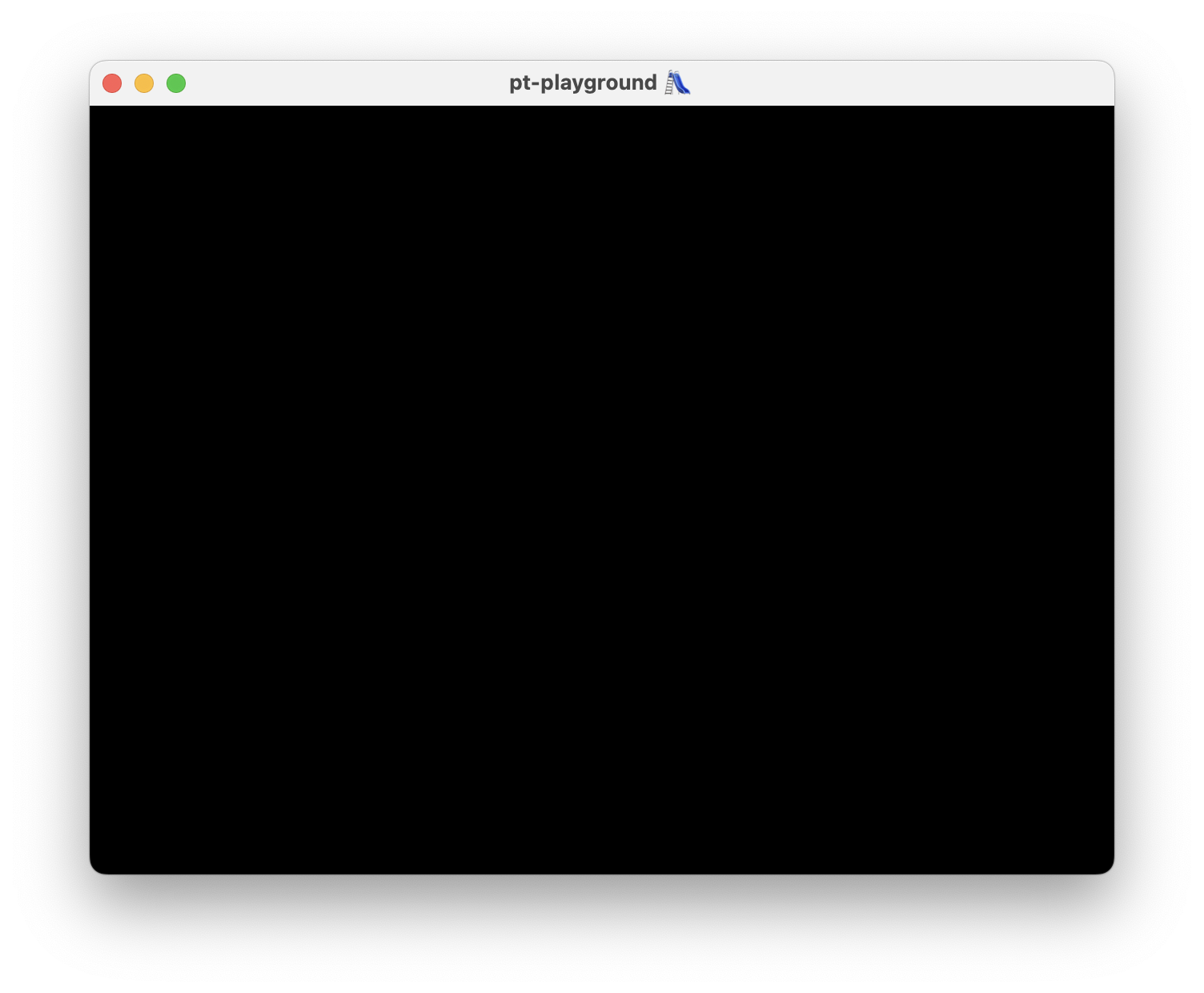# Pathtracer Devlog

This post is a devlog for my pathtracer-playground project. Follow along to see the progress, starting from scratch by opening a simple window.

## Filmic tone mapping and better tone mapping controls

2023-12-03

Commit: 37bfce8

The expose function from the previous entry was not a very good tonemapping curve and was baked into the raytracing shader.

The ACES filmic tone mapping curve is a curve fit of a complex tone mapping algorithm used in the film industry and looks better than the curve used previously.

A simple exposure control multiplier is introduced, defined in terms of stops.

$$\mathrm{exposure} = \frac{1}{2^{\mathrm{stop}}}, \mathrm{stop} \geq 0.$$

The exposure is halved each time the stop is incremented. The Monte Carlo estimator is scaled by the exposure multiplier and passed through the tone mapping function, which yields the final value.

 1 2 3 4 5 6  let stops = f32(postProcessingParams.stops); let exposure = 1f / pow(2f, stops); // tonemapFn is an enumeration with linear = 0, filmic = 1. let tonemapFn = postProcessingParams.tonemapFn; let rgb = expose(tonemapFn, exposure * estimator); 

A linear tone map with stops = 4 yields the following image.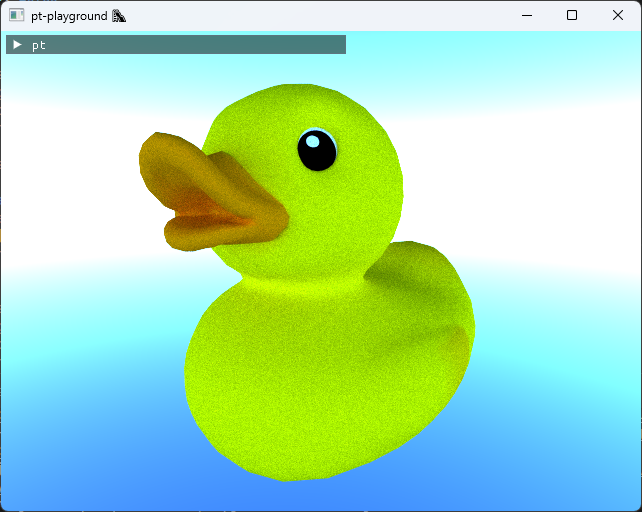Filmic tone map with stops = 4 yields a much better looking image.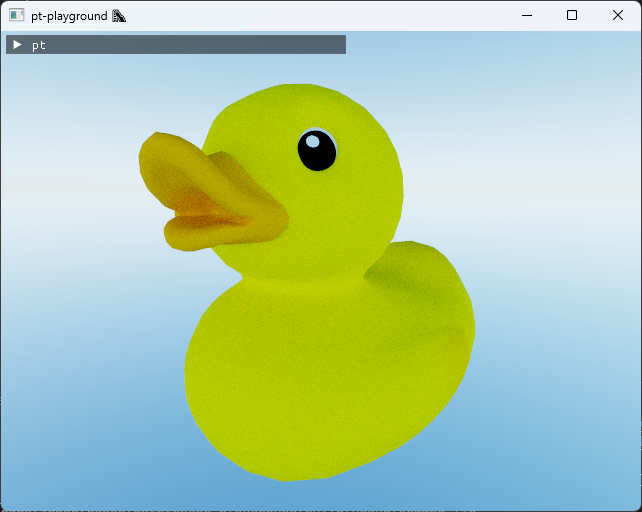## Integrating a physically-based sky model

2023-11-20

Commit: c040325

The Hosek-Wilkie sky model is integrated in the renderer. The model implementation is a straightforward C-port of the hw-skymodel Hosek-Wilkie model implementation.

The model provides physically-based radiance values for any direction in the sky, assuming the viewer is on the ground of the Earth. The model was obtained by doing brute-force simulations of the atmospheric scattering process on Earth, and then fitting the results into a simple model that is much faster to evaluate at runtime.

The sky model parameters, such as the sun’s position, can be updated in real-time. This involves recomputing the values in a look-up table, and uploading them to the GPU. The look-up table values are used to compute the radiance for each ray which scatters towards the sky:

  1 2 3 4 5 6 7 8 9 10 11 12 13 14 15 16 17 18 19 20 21 22 23  for (var bounces = 0u; bounces < NUM_BOUNCES; bounces += 1u) { var intersection: Intersection; if rayIntersectBvh(ray, T_MAX, &intersection) { // ... } else { // Ray missed. Compute sky radiance. let v = ray.direction; let s = skyState.sunDirection; let theta = acos(v.y); let gamma = acos(clamp(dot(v, s), -1f, 1f)); let skyRadiance = vec3f( radiance(theta, gamma, CHANNEL_R), radiance(theta, gamma, CHANNEL_G), radiance(theta, gamma, CHANNEL_B) ); color += throughput * skyRadiance; break; } } 

The model does not return RGB color values, but physical radiance values along each ray. These values are much larger than the [0, 1] RGB color range. The following expose function is used to map the radiance values to a valid RGB color:

 1 2 3  fn expose(v: vec3f, exposure: f32) -> vec3f { return vec3(2.0f) / (vec3(1.0f) + exp(-exposure * v)) - vec3(1.0f); } 

Here is what the full sky dome looks like, rendered about the vertical y-axis.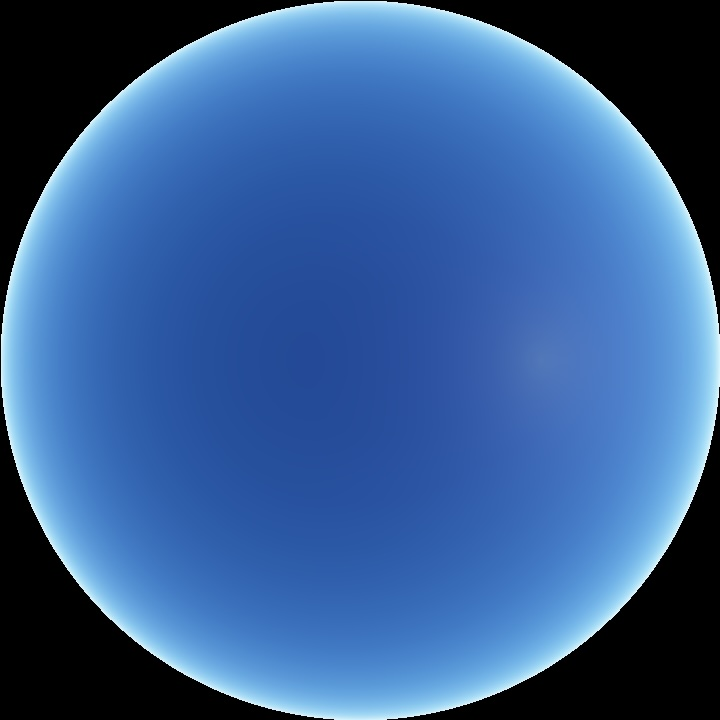The lighting scattering off the duck model also looks distinctly different from before.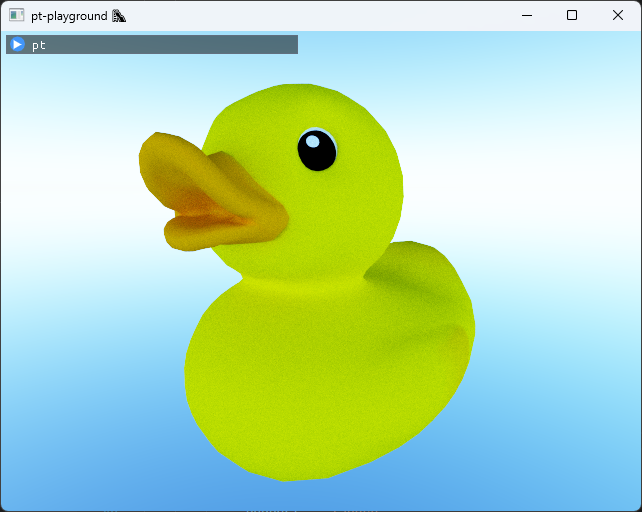## Even better estimator: simple temporal accumulation

2023-11-15

Commit: b10570a

Temporal accumulation is implemented and the sum term is added back to our Monte Carlo estimator:

$$F_n = \frac{1}{n} \sum_{i = 1}^{n} k_{\textrm{albedo}}L_i(\omega_i),$$

1 sample per pixel is accumulated per frame, and our estimator is thus active over multiple frames. If the camera moves, or different rendering parameters are selected, rendering the image starts from scratch.

The duck after 256 samples per pixel: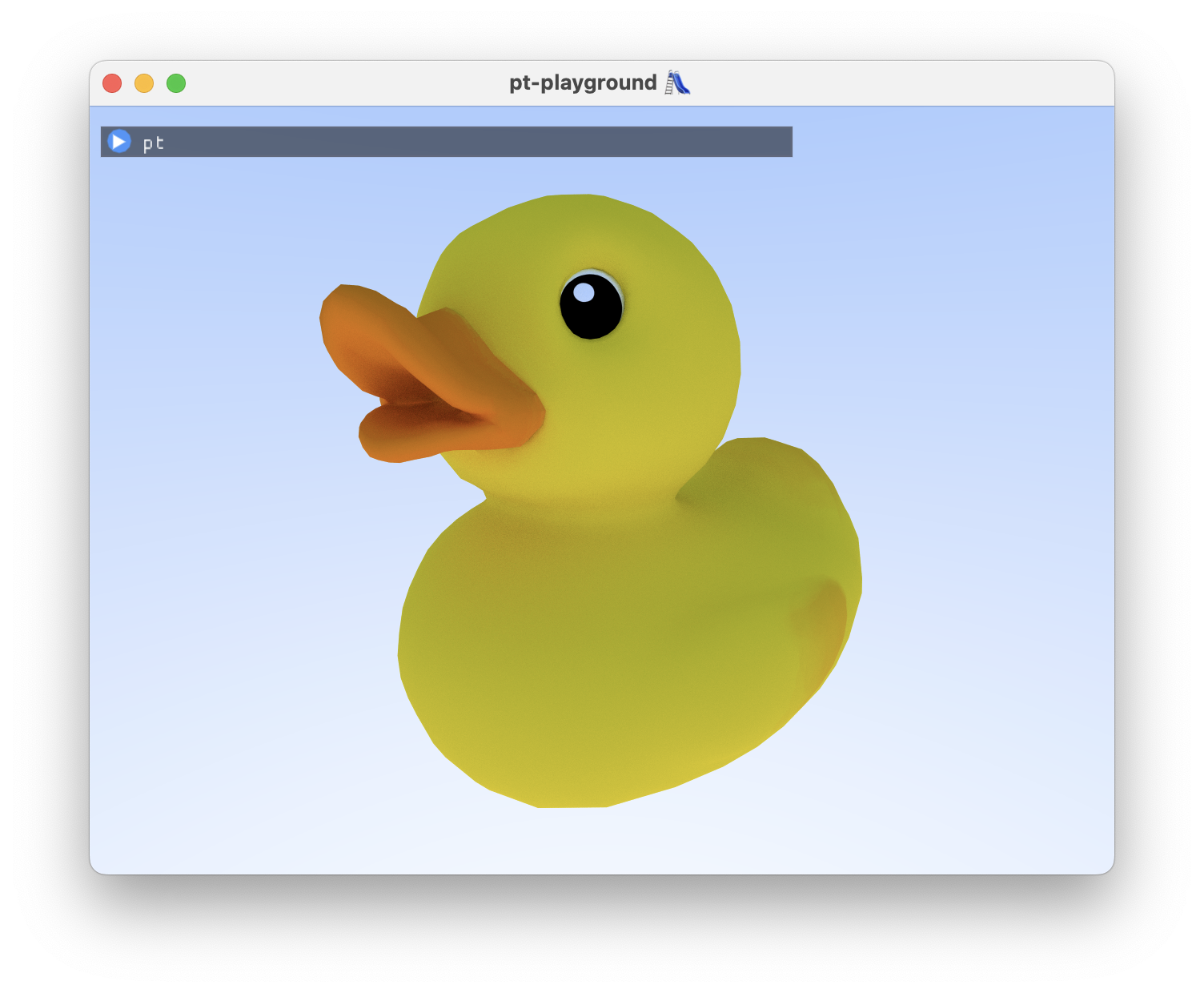## Better estimator: importance sampling surface normal directions

2023-11-14

Commit: 4a76335

Cosine-weighted hemisphere sampling is implemented. The term $$(\omega_i \cdot \mathrm{n})$$ in the rendering equation equals $$\cos \theta$$, where $$\theta$$ is the zenith angle between the surface normal and the incoming ray direction. Directions which are close to perpendicular to the surface normal contribute very little light due to the cosine. It would be better to sample directions close to the surface normal.

The Monte Carlo estimator for non-uniform random variables $$X_i$$ drawn out of probability density function (PDF) $$p(x)$$ is

$$F_n = \frac{1}{n} \sum_{i = 1}^{n} \frac{f(X_i)}{p(X_i)}.$$

Choosing $$p(\omega) = \frac{\cos \theta}{\pi}$$ and, like before, taking just one sample, our estimator becomes:

$$F_1 = \frac{\pi}{\cos \theta} f_{\textrm{lam}}L_i(\omega_i)(\omega_i \cdot \mathrm{n}).$$

We can substitute $$(\omega_i \cdot \mathrm{n}) = \cos \theta$$ and $$f_{\textrm{lam}} = \frac{k_{\textrm{albedo}}}{\pi}$$ to simplify the estimator further:

$$F_1 = k_{\textrm{albedo}}L_i(\omega_i),$$

where our light direction $$\omega_i$$ is now drawn out of the cosine-weighted hemisphere. The code is simplified to just one function, which both samples the cosine-weighted direction and evaluates the albedo:

  1 2 3 4 5 6 7 8 9 10 11 12 13 14 15 16  struct Scatter { wi: vec3f, throughput: vec3f, } fn evalImplicitLambertian(hit: Intersection, rngState: ptr) -> Scatter { let v = rngNextInCosineWeightedHemisphere(rngState); let onb = pixarOnb(hit.n); let wi = onb * v; let textureDesc = textureDescriptors[textureDescriptorIndices[hit.triangleIdx]]; let uv = hit.uv; let albedo = textureLookup(textureDesc, uv); return Scatter(wi, albedo); } 

### Inverse transform sampling the cosine-weighted unit hemisphere.

The exact same recipe that was used for the unit hemisphere can be followed here to obtain rngNextInCosineWeightedHemisphere. While the recipe used is introduced in Physically Based Rendering, the book does not present this calculation.

Choice of PDF

Set probability density to be $$p(\omega) \propto \cos \theta$$.

PDF normalization

Integrate $$p(\omega)$$ over hemisphere H again.

$$\int_H p(\omega) d\omega = \int_{0}^{2 \pi} \int_{0}^{\frac{\pi}{2}} c \cos \theta \sin \theta d\theta d\phi = \frac{c}{2} \int_{0}^{2 \pi} d\phi = 1$$ $$\Longrightarrow c = \frac{1}{\pi}$$

Coordinate transformation

Again, using the result $$p(\theta, , \phi) = \sin \theta p(\omega)$$, obtain

$$p(\theta , \phi) = \frac{1}{\pi} \cos \theta \sin \theta.$$

$$\theta$$’s marginal probability density

$$p(\theta) = \int_{0}^{2 \pi} p(\theta , \phi) d \phi = \int_{0}^{2 \pi} \frac{1}{\pi} \cos \theta \sin \theta d \phi = 2 \sin \theta \cos \theta.$$

$$\phi$$’s conditional probability density

$$p(\phi | \theta) = \frac{p(\theta , \phi)}{p(\theta)} = \frac{1}{2 \pi}$$

Calculate the CDF’s

$$P(\theta) = \int_{0}^{\theta} 2 \cos \theta^{\prime} \sin \theta^{\prime} d \theta^{\prime} = 1 - \cos^2 \theta$$ $$P(\phi | \theta) = \int_{0}^{\phi} \frac{1}{2 \pi} d\phi^{\prime} = \frac{\phi}{2 \pi}$$

Inverting the CDFs in terms of u

$$\cos \theta = \sqrt{1 - u_1} = \sqrt{u_1}$$

$$\phi = 2 \pi u_2$$

where $$u_1 = 1 - u_1$$ is substituted again. Converting $$\theta$$, $$\phi$$ back to Cartesian coordinates, note that $$z = \cos \theta = \sqrt{u_1}$$.

$$x = \sin \theta \cos \phi = \sqrt{1 - u_1} \cos(2 \pi u_2)$$ $$y = \sin \theta \sin \phi = \sqrt{1 - u_1} \sin(2 \pi u_2)$$ $$z = \sqrt{u_1}$$

In code:

  1 2 3 4 5 6 7 8 9 10 11 12 13  fn rngNextInCosineWeightedHemisphere(state: ptr) -> vec3f { let u1 = rngNextFloat(state); let u2 = rngNextFloat(state); let phi = 2f * PI * u2; let sinTheta = sqrt(1f - u1); let x = cos(phi) * sinTheta; let y = sin(phi) * sinTheta; let z = sqrt(u1); return vec3(x, y, z); }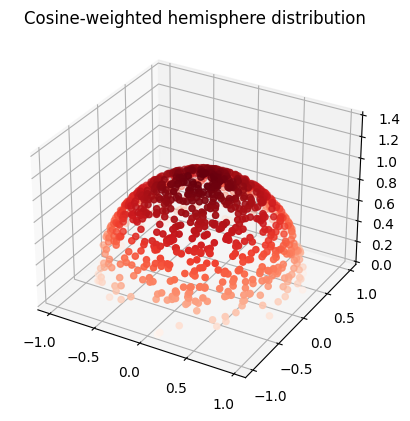Looking at our duck, before: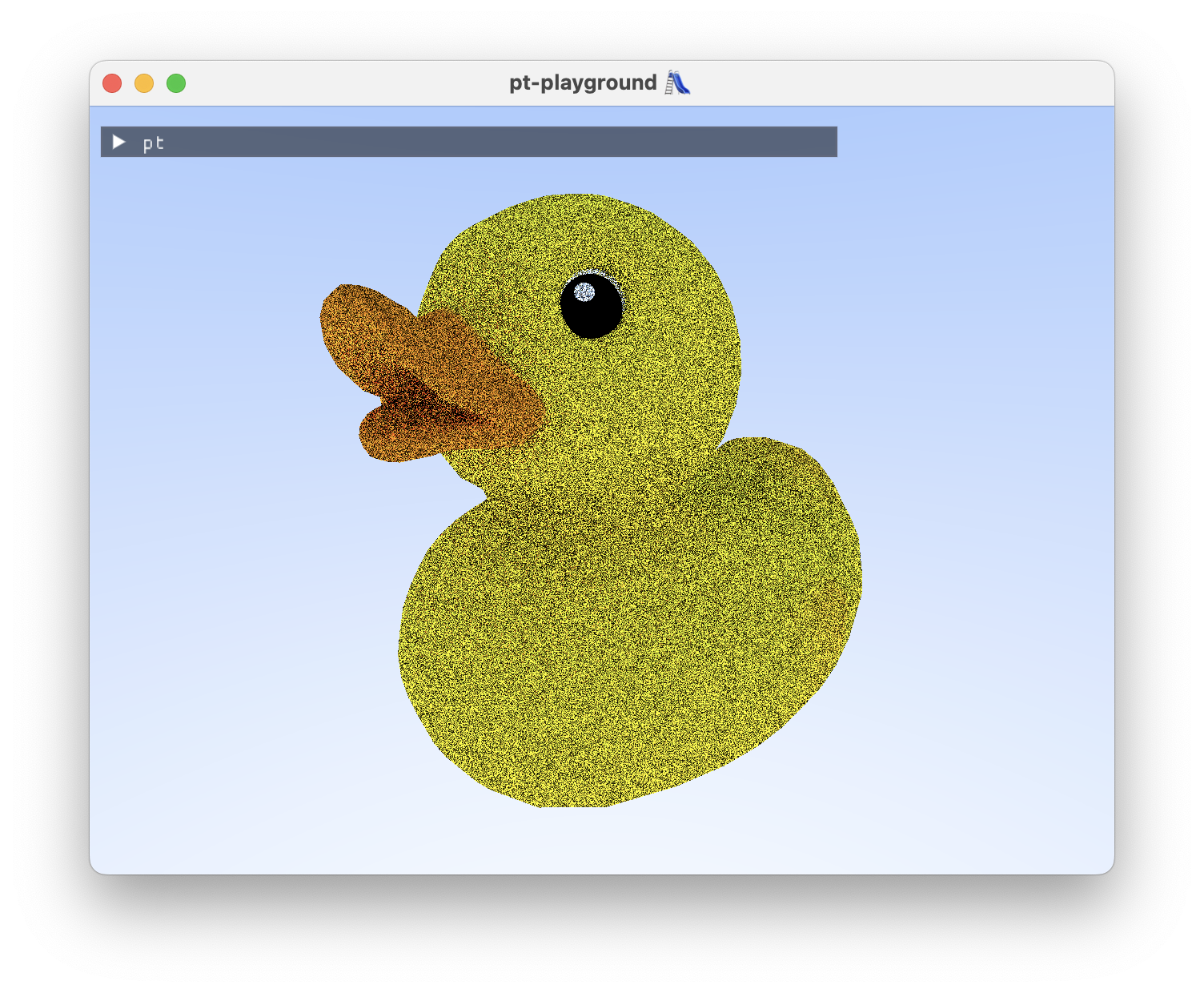And after, with cosine-weighted hemisphere sampling: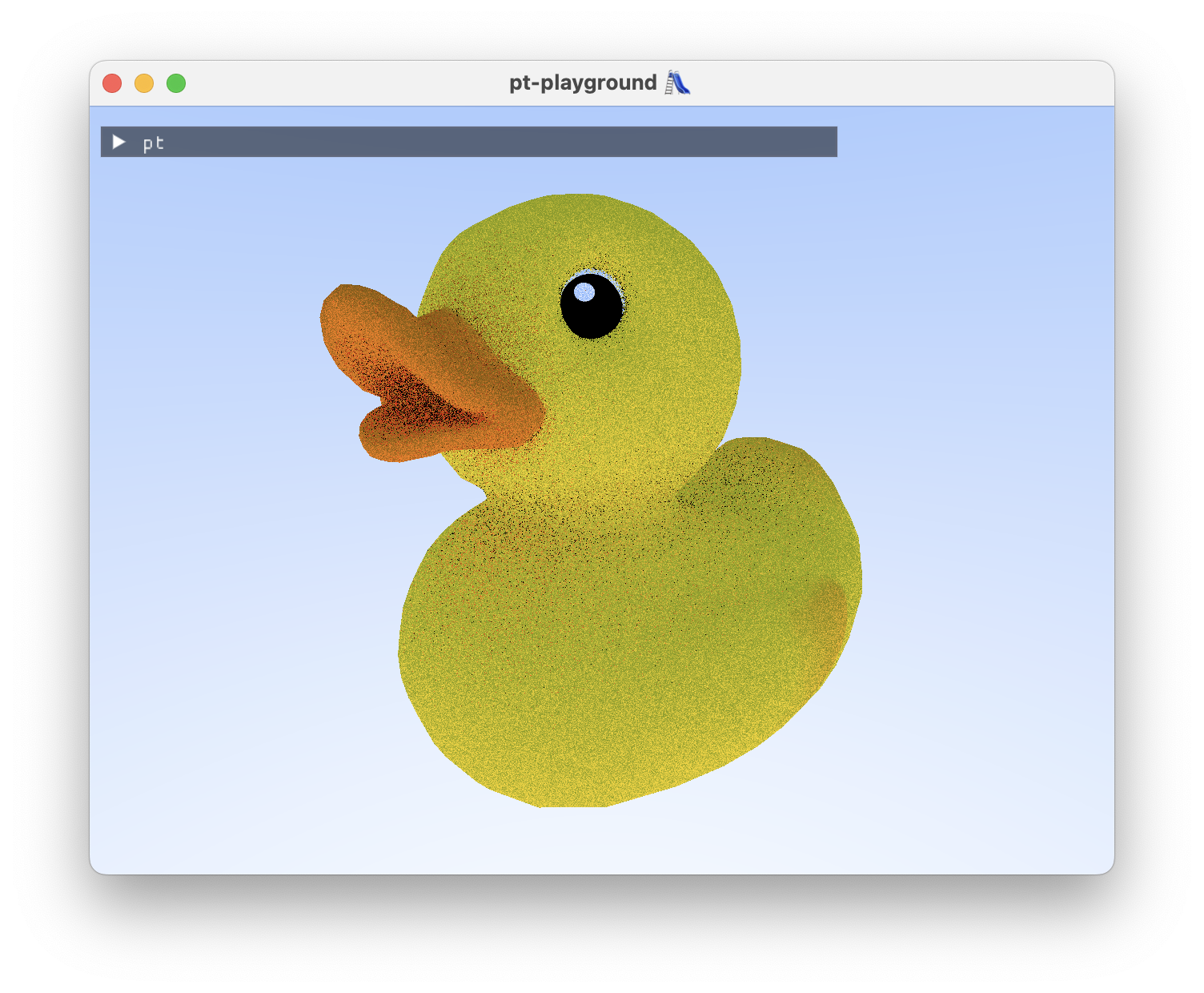## The first physically-based pathtracer

2023-11-13

Commit: c83b83e

A simple physically-based pathtracer is implemented. ✨ The rendering equation is solved using the following Monte Carlo estimator:

$$F_1 = 2 \pi f_r(\mathrm{x_i},\omega_i)L_i(\mathrm{x_i},\omega_i)(\omega_i\cdot\mathrm{n}),$$

where $$2 \pi$$ is the surface area of the unit hemisphere, $$f_r$$ is the bidirectional reflectance distribution function (BRDF), $$\omega_i$$ is the incoming ray direction, and $$L_i$$ is the incoming radiance along $$\omega_i$$. The estimator is based on the Monte Carlo estimator for $$\int_{a}^{b} f(x) dx$$, where the random variable is drawn from a uniform distribution:

$$F_n = \frac{b-a}{n} \sum_{i=1}^{n} f(X_i),$$

where $$n=1$$ is set for now and a surface area is used instead of the 1-dimensional range.

For simplicity, only the Lambertian BRDF is implemented at this stage, with $$f_{\textrm{lam}}=\frac{k_{\textrm{albedo}}}{\pi}$$. Drawing inspiration from Crash Course in BRDF implementation, a pair of functions are implemented:

  1 2 3 4 5 6 7 8 9 10 11 12  fn sampleLambertian(hit: Intersection, rngState: ptr) -> vec3f { let v = rngNextInUnitHemisphere(rngState); let onb = pixarOnb(hit.n); return onb * v; } fn evalLambertian(hit: Intersection, wi: vec3f) -> vec3f { let textureDesc = textureDescriptors[textureDescriptorIndices[hit.triangleIdx]]; let uv = hit.uv; let albedo = textureLookup(textureDesc, uv); return albedo * FRAC_1_PI * max(EPSILON, dot(hit.n, wi)); } 

### Inverse transform sampling the unit hemisphere.

To obtain rngNextInUnitHemisphere, we can follow a recipe introduced in Physically Based Rendering. Here’s a quick summary of the calculation, which is also partially in the book.

The recipe

In order to do Monte Carlo integration, you will need to do inverse transform sampling with your selected probability density function (PDF). Here’s the general steps that you need to take to arrive at a function that you can use to draw sampels from.

1. Choose the probability density function.
2. If the density function is multidimensional, you can compute the marginal density (integrate out one variable), and the conditional density for the other variable.
3. Calculate the cumulative distribution function (CDF).
4. Invert the CDFs in terms of the canonical uniform random variable, $$u$$.

Choice of PDF

Uniform probability density over all directions $$\omega$$, $$p(\omega) = c$$.

PDF normalization

The integral over the hemisphere H yields the surface area of a hemisphere:

$$\int_{H} c d \omega = 2 \pi c = 1$$

$$c = \frac{1}{2 \pi}$$

Coordinate transformation

Use the result that $$p(\theta, , \phi) = \sin \theta p(\omega)$$ to obtain

$$p(\theta , \phi) = \frac{\sin \theta}{2 \pi}$$

$$\theta$$’s marginal probability density

$$p(\theta) = \int_{0}^{2 \pi} p(\theta , \phi) d \phi = \int_{0}^{2 \pi} \frac{\sin \theta}{2 \pi} d \phi = \sin \theta$$

$$\phi$$’s conditional probability density

In general, $$\phi$$’s probability density would be conditional on $$\theta$$ after computing $$\theta$$’s marginal density.

$$p(\phi | \theta) = \frac{p(\theta , \phi)}{p(\theta)} = \frac{1}{2 \pi}$$

Calculate the CDF’s

$$P(\theta) = \int_{0}^{\theta} \sin \theta^{\prime} d\theta^{\prime} = 1 - \cos \theta$$

$$P(\phi | \theta) = \int_{0}^{\phi} \frac{1}{2 \pi} d\phi^{\prime} = \frac{\phi}{2 \pi}$$

Inverting the CDFs in terms of u

$$\theta = \cos^{-1}(1 - u_1)$$

$$\phi = 2 \pi u_2$$

$$u_1 = 1 - u_1$$ can be substituted as it is just another canonical uniform distribution, to obtain

$$\theta = \cos^{-1}(u_1)$$

$$\phi = 2 \pi u_2$$

Convert $$\theta$$ and $$\phi$$ back to Cartesian coordinates

$$x = \sin \theta \cos \phi = \sqrt{1 - u_1^2} \cos(2 \pi u_2)$$ $$y = \sin \theta \sin \phi = \sqrt{1 - u_1^2} \sin(2 \pi u_2)$$ $$z = u_1$$

where we used the identity $$\sin \theta = \sqrt{1 - \cos^2 \theta}$$. In code:

  1 2 3 4 5 6 7 8 9 10 11 12 13  fn rngNextInUnitHemisphere(state: ptr) -> vec3f { let u1 = rngNextFloat(state); let u2 = rngNextFloat(state); let phi = 2f * PI * u2; let sinTheta = sqrt(1f - u1 * u1); let x = cos(phi) * sinTheta; let y = sin(phi) * sinTheta; let z = u1; return vec3(x, y, z); } 

It generates unit vectors, distributed uniformly in a hemisphere about the z axis.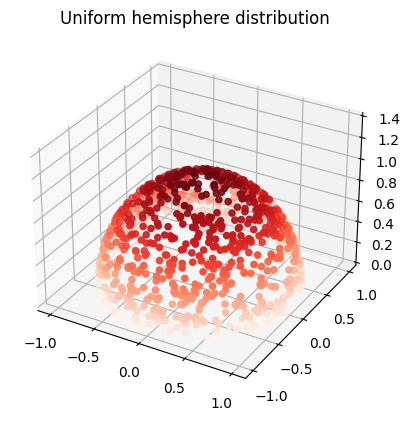The functions are used in the path-tracing loop as follows:

  1 2 3 4 5 6 7 8 9 10 11 12 13 14 15 16 17 18 19 20 21 22 23 24 25  const UNIFORM_HEMISPHERE_MULTIPLIER = 2f * PI; fn rayColor(primaryRay: Ray, rngState: ptr) -> vec3f { var ray = primaryRay; var color = vec3(0f); var throughput = vec3(1f); for (var bounces = 0u; bounces < NUM_BOUNCES; bounces += 1u) { var intersection: Intersection; if rayIntersectBvh(ray, T_MAX, &intersection) { let p = intersection.p; let scatterDirection = sampleLambertian(intersection, rngState); let scatterThroughput = UNIFORM_HEMISPHERE_MULTIPLIER * evalLambertian(intersection, scatterDirection); ray = Ray(p, scatterDirection); throughput *= scatterThroughput; } else { // compute sky color ... break; } } return color; } 

We can now pathtrace our duck. If the noise on the duck exhibits a grid-like pattern, you should open the full-size image as the website scales the images down.Now that real work is being done in the shader, the performance of Sponza on my M2 Macbook Air is starting to become punishing. Framerates are in the 1-10 FPS range with the depicted image sizes.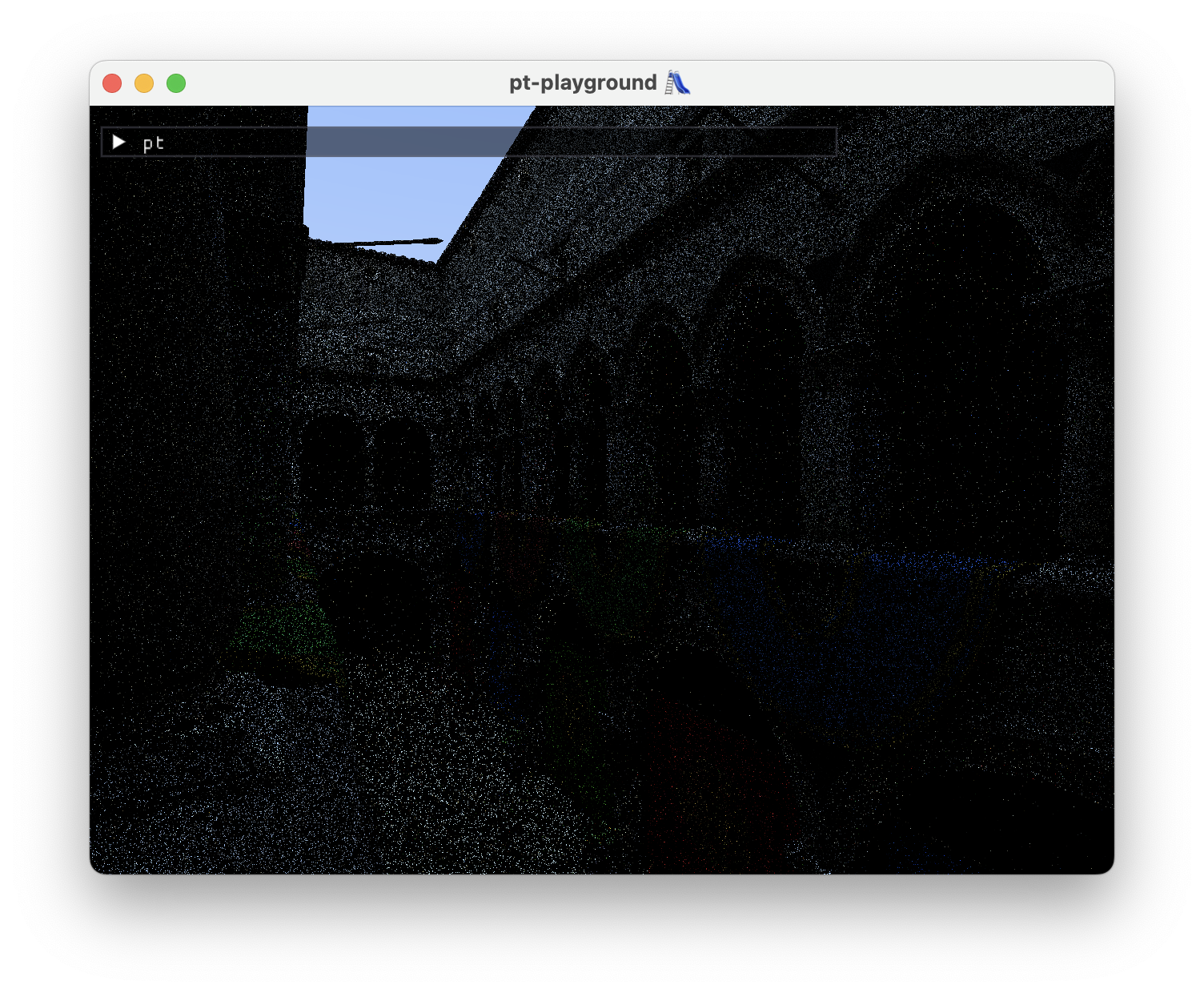## Simple texture support 🖼️

2023-11-10

Commit: 8062be7

Initial textures support is added. The “base color texture” from the glTF “pbr metallic roughness” material is loaded and passed over to the GPU. The same storage buffer approach from my Weekend raytracing with wgpu, part 2 post is used for texture lookup on the GPU.

 1 2 3 4 5 6 7 8  // Each triangle is associated with an index to a texture descriptor, a // placeholder for a texture on the GPU. @group(2) @binding(4) var textureDescriptorIndices: array; // A texture descriptor contains an offset into the texture buffer, as // well as the texture dimensions. @group(2) @binding(5) var textureDescriptors: array; // The texture buffer contains textures from all meshes, appended end to end. @group(2) @binding(6) var textures: array; 

On ray-triangle intersection, the model vertex UV coordinates are interpolated and the color is computed from the corresponding texel.

  1 2 3 4 5 6 7 8 9 10 11 12 13 14 15 16 17 18 19 20 21  if rayIntersectBvh(ray, T_MAX, &intersection, &triangleIdx) { let textureDesc = textureDescriptors[textureDescriptorIndices[triangleIdx]]; let b = intersection.b; let uvs = texCoords[triangleIdx]; let uv = b * uvs + b * uvs + b * uvs; color = textureLookup(textureDesc, uv); } fn textureLookup(desc: TextureDescriptor, uv: vec2f) -> vec3f { let u = clamp(uv.x, 0f, 1f); let v = clamp(uv.y, 0f, 1f); let j = u32(u * f32(desc.width)); let i = u32(v * f32(desc.height)); let idx = i * desc.width + j; let rgba = textures[desc.offset + idx]; return vec3f(f32(rgba & 0xffu), f32((rgba >> 8u) & 0xffu), f32((rgba >> 16u) & 0xffu)) / 255f; } 

The duck model from earlier looks more like a rubber duck now.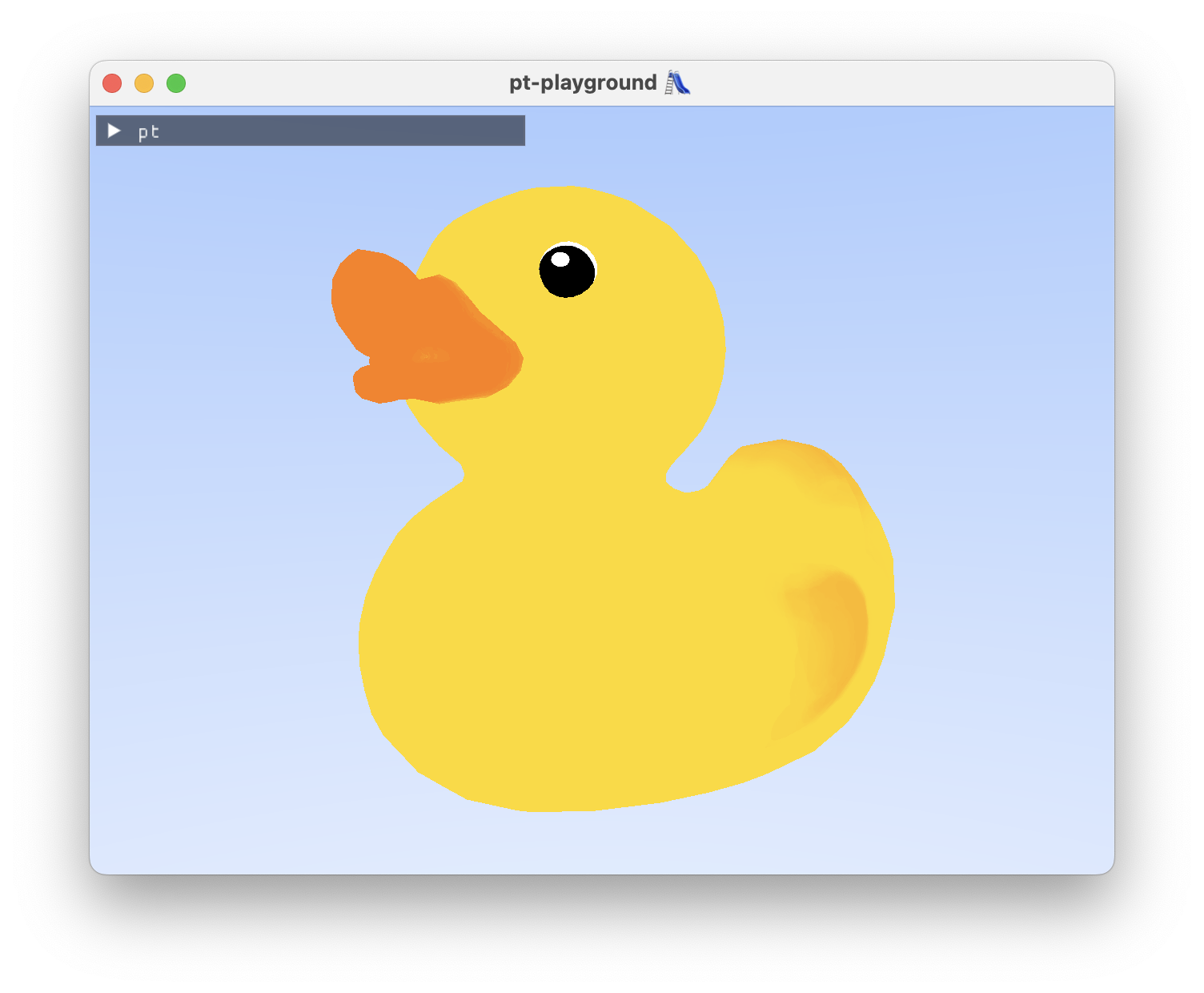Sponza looks a bit rough at this stage. Two things need work.

• The plants in the foreground need transparency support. On intersection, the transparency of the pixel also needs to be accounted for the ray to intersect the triangle.
• There is an issue with the textures on the ground and in some places on the walls being stretched out.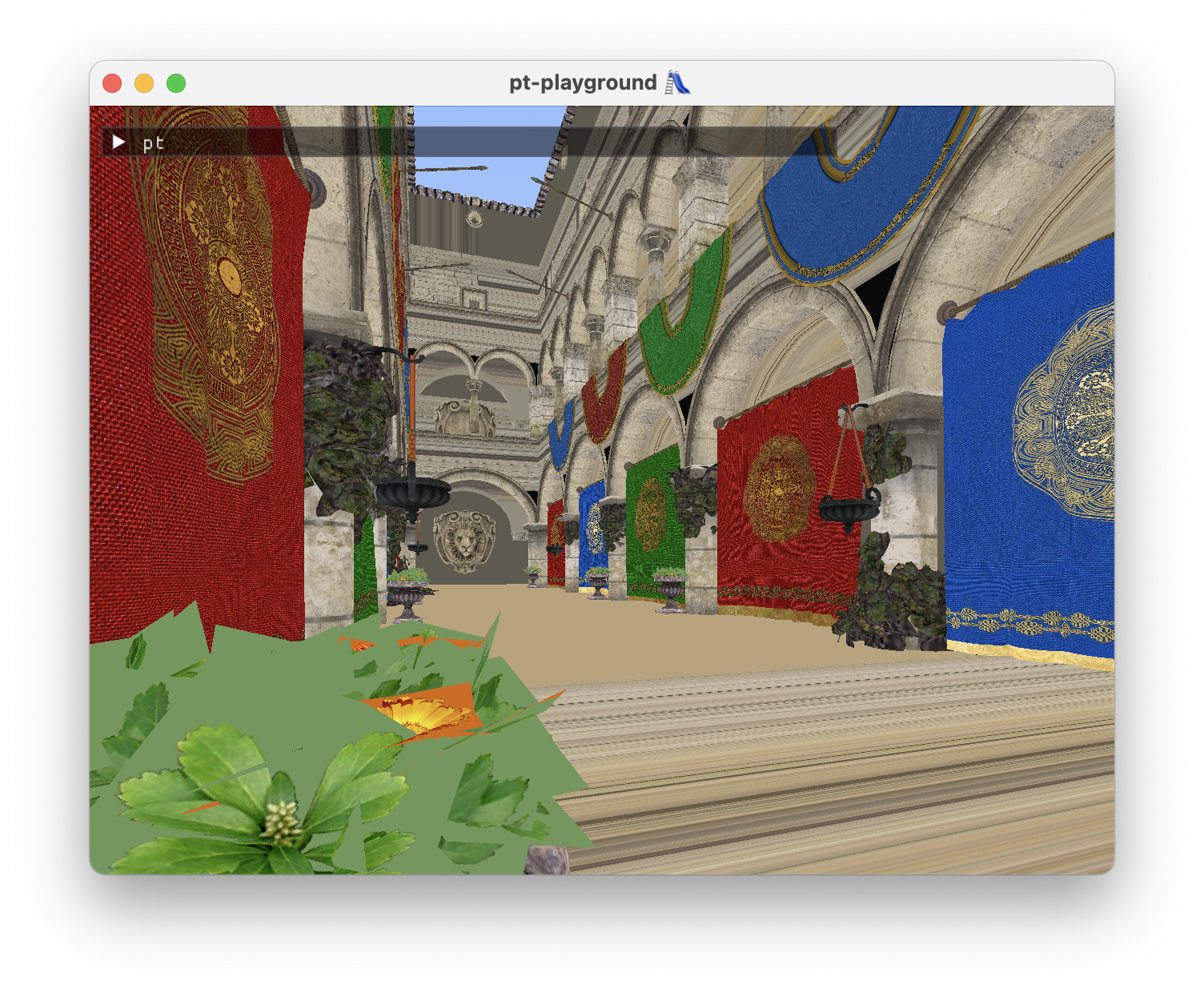The stretched textures are likely due to a problem with UV coordinate interpolation. Single colors and suspicious red-black color gradients can be seen when coloring the model according to the triangles’ interpolated UV coordinates.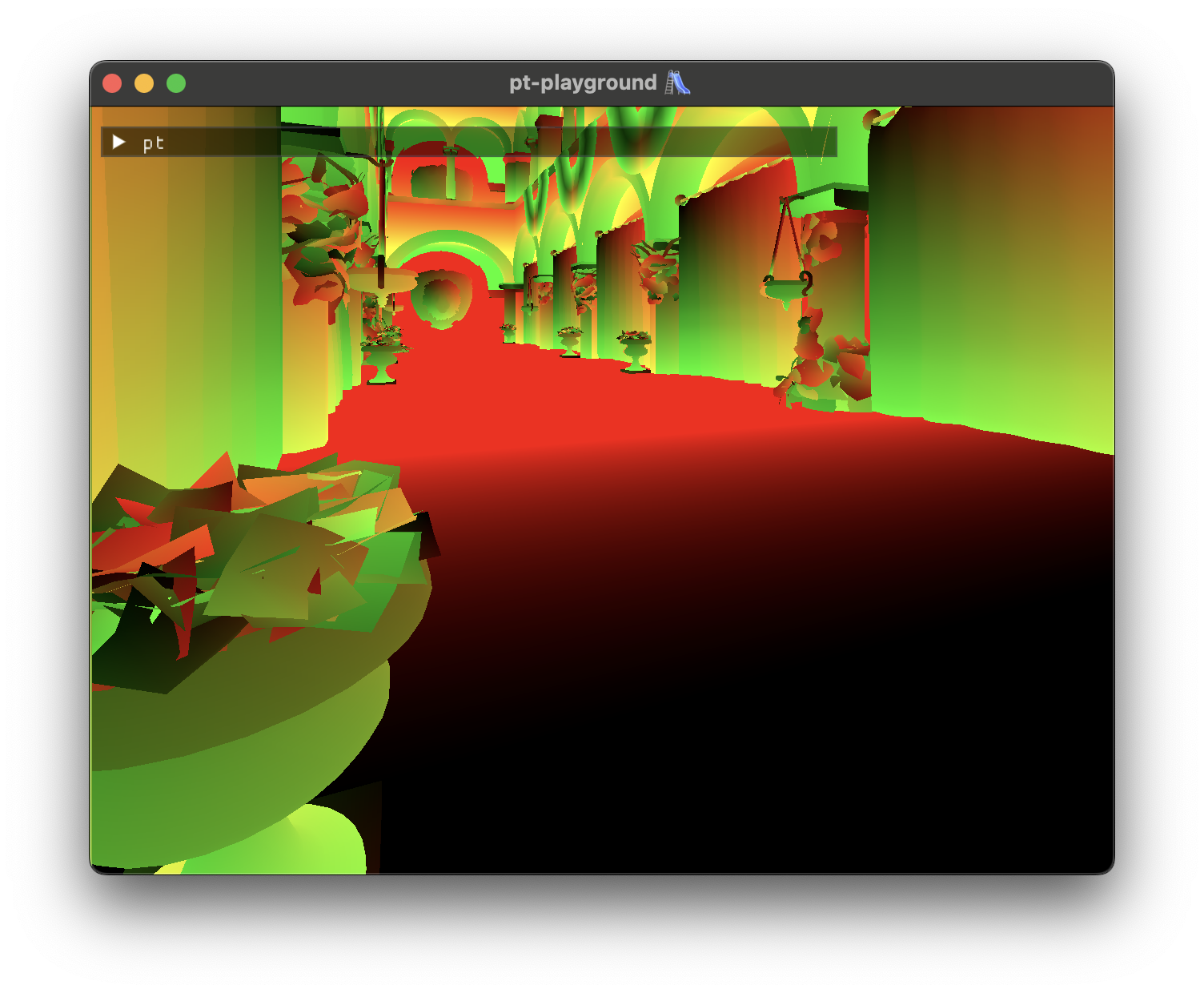## Interpolated normals, texture coordinates, and GPU timers

2023-11-09

Commit: 82208c1

GPU timers

Timing the render pass on the device timeline allows us to keep track of how long rendering the scene actually takes on the GPU, no matter what I do on the CPU side. The timings are displayed in the UI as a moving average.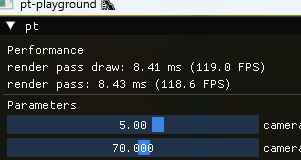My 3080 RTX currently runs the Sponza atrium scene (see below) at roughly 120 FPS at fullscreen resolutions.

Enabling allow-unsafe-apis

WebGPU timestamp queries are disabled by default due to timings being potentially usable for client fingerprinting. Using the timestamp queries requires toggling “allow-unsafe-apis” (normally toggled via Chrome’s settings) using the WebGPU native’s struct extension mechanism:

  1 2 3 4 5 6 7 8 9 10 11 12 13 14 15 16  const char* allowUnsafeApisToggle = "allow_unsafe_apis"; WGPUDawnTogglesDescriptor instanceToggles = { .chain = WGPUChainedStruct{ .next = nullptr, .sType = WGPUSType_DawnTogglesDescriptor, }, .enabledTogglesCount = 1, .enabledToggles = &allowUnsafeApisToggle, .disabledTogglesCount = 0, .disabledToggles = nullptr, }; const WGPUInstanceDescriptor instanceDesc{ .nextInChain = &instanceToggles.chain, }; const WGPUInstance instance = wgpuCreateInstance(&instanceDesc); 

The model surface normals and texture coordinates are loaded from the model. The model now exposes arrays of vertex attributes:

  1 2 3 4 5 6 7 8 9 10 11 12 13 14 15 16 17  class GltfModel { public: GltfModel(std::filesystem::path gltfPath); std::span positions() const { return mPositions; } std::span normals() const { return mNormals; } std::span texCoords() const { return mTexCoords; } std::span baseColorTextureIndices() const { return mBaseColorTextureIndices; } std::span baseColorTextures() const { return mBaseColorTextures; } private: ... }; 

Normals and texture coordinates are interpolated at the intersection point using the intersection’s barycentric coordinates:

 1 2 3 4 5 6 7  if rayIntersectBvh(ray, T_MAX, &intersection, &triangleIdx) { let b = intersection.b; let tnorms = normals[triangleIdx]; let n = b * tnorms + b * tnorms + b * tnorms; color = 0.5f * (vec3f(1f, 1f, 1f) + n); } 

Here’s the famous Sponza atrium scene demonstrating the smoothly interpolated normals: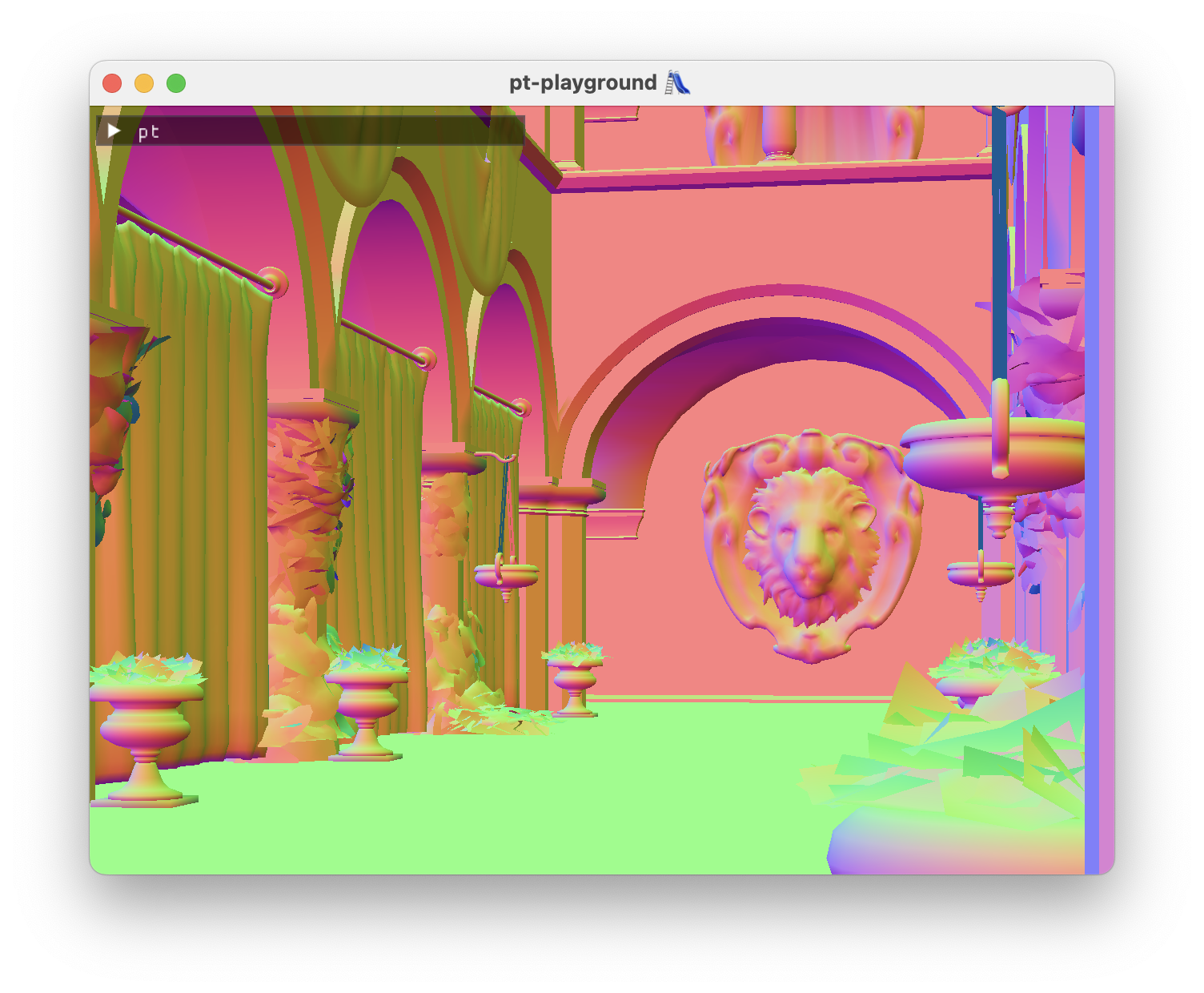2023-11-08

Commit: 1706265

The glTF model loader now loads textures, and the base color textures are exposed in the model:

  1 2 3 4 5 6 7 8 9 10 11 12 13 14 15  class GltfModel { public: GltfModel(std::filesystem::path gltfPath); std::span triangles() const { return mTriangles; } std::span baseColorTextureIndices() const { return mBaseColorTextureIndices; } std::span baseColorTextures() const { return mBaseColorTextures; } private: ... }; 

Each triangle has a base color texture index, and it can be used to index into the array of base color textures. Textures are just arrays of floating point values.

A new textractor executable (short for texture extractor 😄) is added. It loads a GLTF model, and then dumps each loaded texture into a .ppm file. This way, it can be visually inspected that the textures have been loaded correctly. For instance, here is the duck model’s base_color_texture_0:## Camera and UI interaction

2023-11-07

Commit: 1593e8a

In order to work with arbitrary 3d models, camera positions and parameters should not be hard-coded. To kickstart scene interaction, two things are implemented:

• A “fly camera”, which allows you to move around and pan the scene around using the mouse.
• A UI integration, and a panel which allows you to edit the camera’s speed, and vfov.

The UI panel also prints out scene debug information:

 1 2 3  Scene camera position: (8.00, 2.80, -8.30) root centroid: (0.13, 0.87, -0.04) 

A bug with the model loader’s model transform meant that the duck was hundreds of units away from the camera vertically. Without the debug UI, it was hard to tell whether I just couldn’t find the model in space, or whether the camera controller had a problem.
## Raytracing triangles on the GPU 🎉

2023-11-06

Commit: e950ea8

Today’s achievement: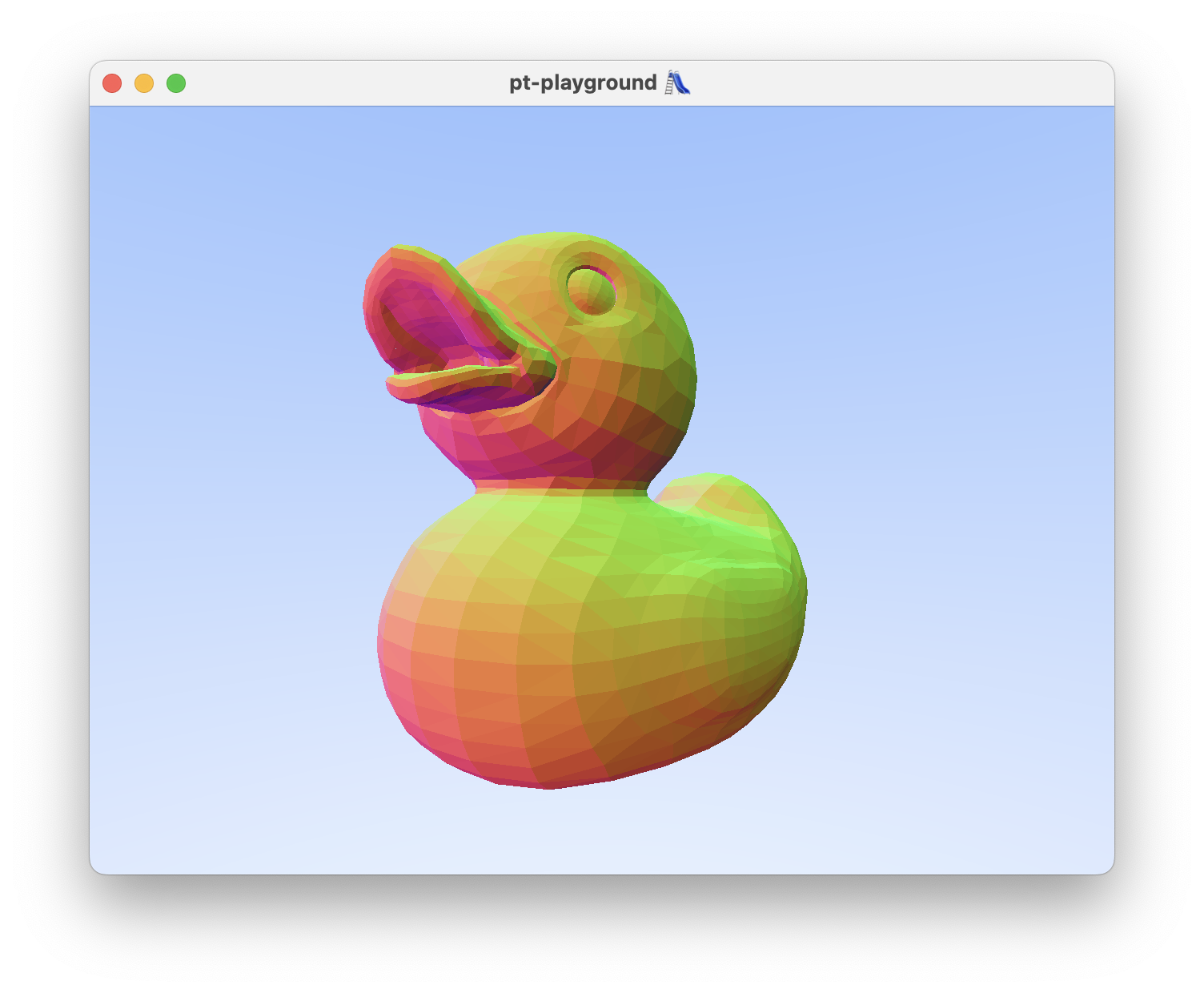The BVH and all intersection testing functions are ported to the shader. The rays from the previous devlog entry are now directly used against the BVH.

Porting the BVH to the GPU involved adjusting the memory layout of a number of structs. Triangles, Aabbs, and the BvhNodes all have vectors in them which need to be 16-byte aligned in memory. This was achieved by padding the structs out. E.g. BvhNode,

  1 2 3 4 5 6 7 8 9 10 11 12 13 14 15 16 17 18 19  -struct BvhNode -{ - Aabb aabb; // offset: 0, size: 24 - union - { - std::uint32_t trianglesOffset; - std::uint32_t secondChildOffset; - }; // offset: 24, size: 4 - std::uint16_t triangleCount; // offset: 28, size: 2 - std::uint16_t splitAxis; // offset: 30, size: 2 -}; +struct BvhNode +{ + Aabb32 aabb; // offset: 0, size: 32 + std::uint32_t trianglesOffset; // offset: 32, size: 4 + std::uint32_t secondChildOffset; // offset: 36, size: 4, + std::uint32_t triangleCount; // offset: 40, size: 4 + std::uint32_t splitAxis; // offset: 44, size: 4 +}; 

I also tried using AI (GitHub Copilot) to translate some of the hundreds of lines of intersection testing code to WGSL. It worked well in the sense that only certain patterns of expression had to be fixed. I likely avoided errors that I would have introduced manually porting the code over.

🤓 TIL about the @must_use tag in WGSL. It’s used as an annotation for functions which return values and don’t have side effects. Not using the function in assignment or an expression becomes a compile error.

 1 2 3 4  @must_use fn rayPointAtParameter(ray: Ray, t: f32) -> vec3f { return ray.origin + t * ray.direction; } 

## Raytracing spheres on the GPU

2023-11-04

Commit: 16f6255

Before attempting to raytrace triangles on the GPU, first I need to demonstrate that the camera actually works in the shader.

To do so, a simple raytracing scenario is first implemented. An array of spheres, stored directly in the shader is added. Ray-sphere intersection code is copied over from my weekend-raytracer-wgpu project. The spheres are colored according to their surface normal vector. The spheres are visible and now I know that the camera works!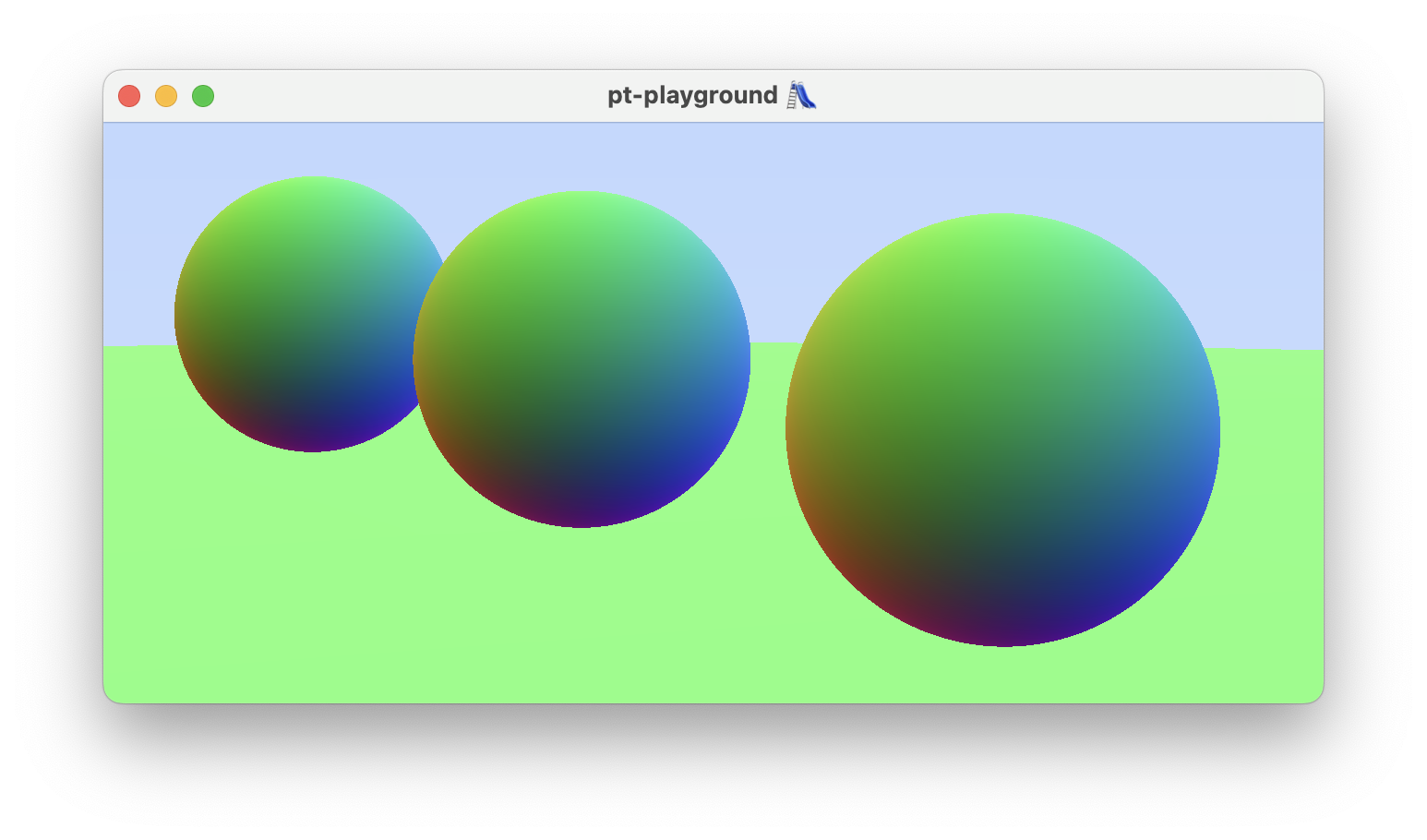The camera and the frame data struct are bundled together into on uniform buffer.

  1 2 3 4 5 6 7 8 9 10 11 12 13 14 15 16 17  struct RenderParams { frameData: FrameData, camera: Camera, } struct FrameData { dimensions: vec2u, frameCount: u32, } struct Camera { eye: vec3f, lowerLeftCorner: vec3f, horizontal: vec3f, vertical: vec3f, lensRadius: f32, } 

I used WGSL Offset Computer, a great online resource for enumerating and drawing the memory layout of your WGSL structs, as a sanity check that my memory layout for renderParams was correct.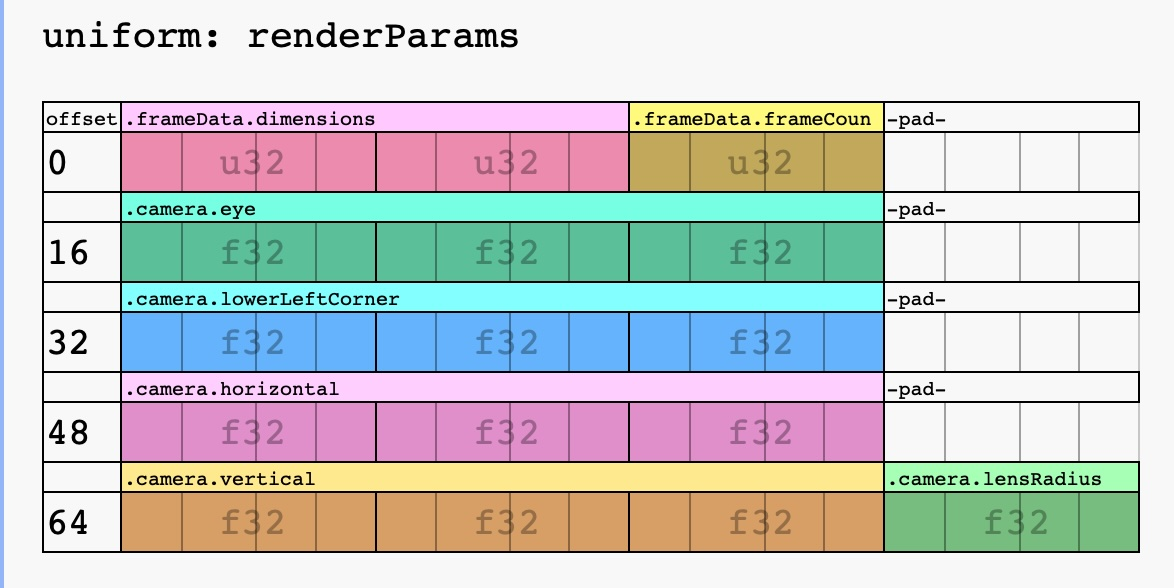## Hello, bounding volume hierarchies

2023-11-02

Commit: 624e5ea

This entry marks the first step towards being able to shoot rays at complex triangle geometry. A bounding volume hierarchy (BVH) is introduced (common/bvh.hpp) and tested.

The test (tests/bvh.cpp) intersects rays with the BVH, and uses a brute-force test against the full array of triangles as the ground truth. The intersection test result (did intersect or did not) and the intersection point, in the case of intersection, must agree for all camera rays in order for the test to pass.

As an additional end-to-end sanity check, a new executable target (bvh-visualizer) is introduced. Primary camera rays are generated and the number of BVH nodes visited per ray is visualized as an image.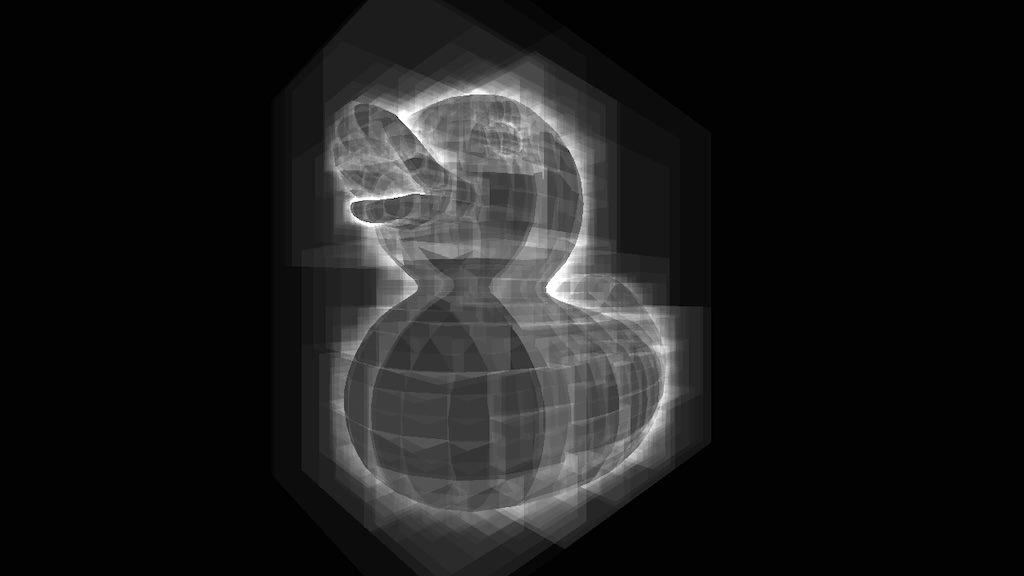## GpuBuffer

2023-11-01

Commit: 0a826c4

Not a lot of visible progress, but GpuBuffer was introduced (in gpu_buffer.hpp) to significantly reduce the amount of boilerplate due to buffers & bind group descriptors:

Showing 5 changed files with 254 additions and 275 deletions.

On another note, used immediately invoked lambda functions in a constructor initializer for the first time today:

  1 2 3 4 5 6 7 8 9 10 11 12 13  Renderer::Renderer(const RendererDescriptor& rendererDesc, const GpuContext& gpuContext) : frameDataBuffer(...), pixelBuffer( gpuContext.device, "pixel buffer", WGPUBufferUsage_CopyDst | WGPUBufferUsage_Storage, [&rendererDesc]() -> std::size_t { const Extent2i largestResolution = rendererDesc.maxFramebufferSize; const std::size_t numPixels = static_cast(largestResolution.x * largestResolution.y); return sizeof(glm::vec3) * numPixels; }()), ... 

It’s busy looking, but it enables you to do actual work in the constructor initializer. Doing all the work in the constructor initializers like this could make move constructors unnecessary.

## GPU-generated noise

2023-10-31

Commit: 645c09c

The major highlights of the day include generating noise on the GPU and displaying it, as well as cleaning up the main function using a new Window abstraction.

Window

A simple Window abstraction is introduced to automate GLFW library and window init and cleanup. main does not yet have an exception handler, but this abstraction is a step closer to ensuring all resources are cleaned up even when exceptions occur.

GPU noise

Noise is generated on the GPU using the following functions.

  1 2 3 4 5 6 7 8 9 10 11 12 13  fn rngNextFloat(state: ptr) -> f32 { rngNextInt(state); return f32(*state) / f32(0xffffffffu); } fn rngNextInt(state: ptr) { // PCG random number generator // Based on https://www.shadertoy.com/view/XlGcRh let oldState = *state + 747796405u + 2891336453u; let word = ((oldState >> ((oldState >> 28u) + 4u)) ^ oldState) * 277803737u; *state = (word >> 22u) ^ word; } 

Each frame,

1. rngNextFloat is used in a compute shader to generate RGB values
2. RGB values are stored in a pixel buffer
3. The pixel buffer is mapped to the full screen quad from the previous day.

This approach is a bit more complex than necessary, and could just be done in the fragment shader.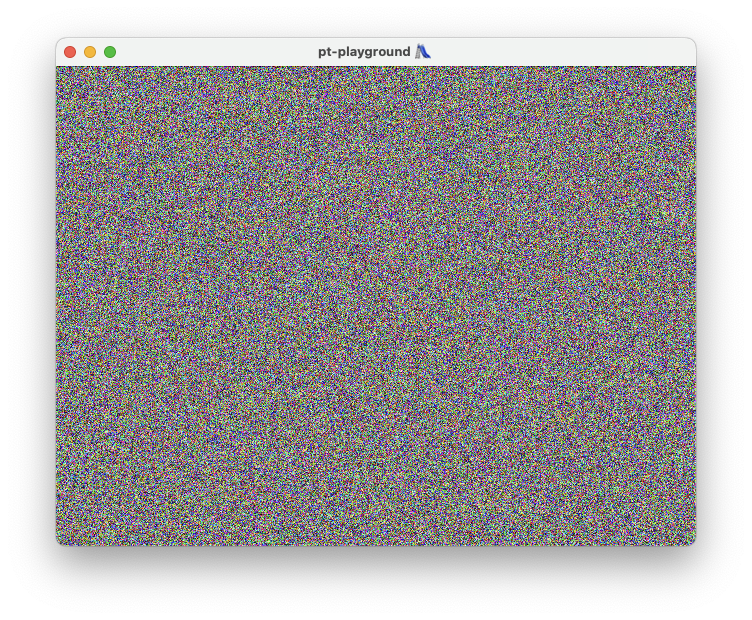2023-10-30

Commit: 51387d7

After 1000 lines of WebGPU plumbing code, the first pair of triangles is displayed on the screen in the shape of a fullscreen quad, and the UV coordinates are displayed as a color.

The pt executable contains

• A GpuContext for initializing the WebGPU instance, surface, adapter, device, queue, and the swap chain.
• A distinct Renderer which initializes a render pipeline, a uniform and vertex buffer, using the GpuContext.

The renderer draws to the screen using a fullscreen quad. For now, the texture coordinates are displayed as colors.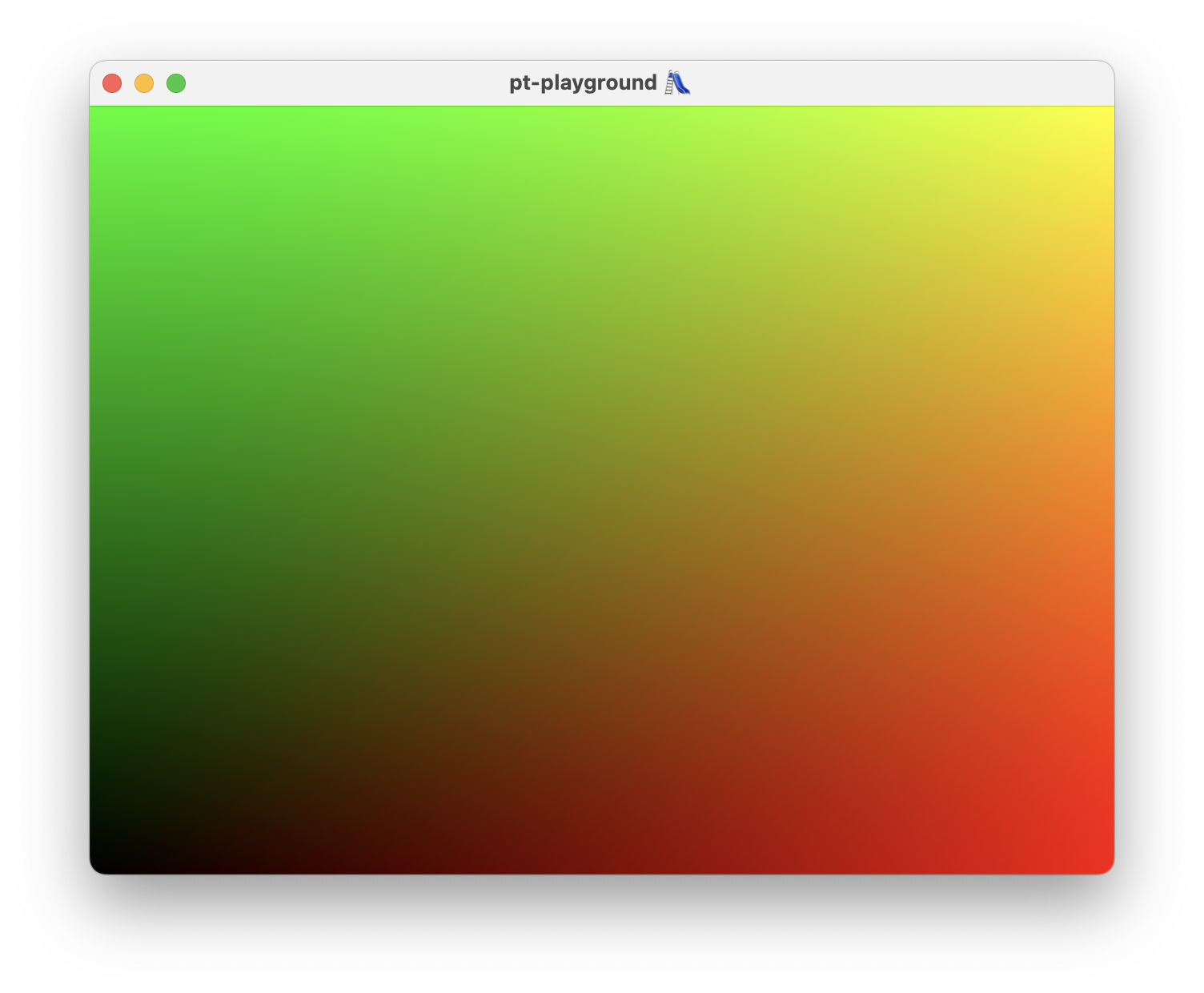## Hello, GLFW!

2023-10-29

Commit: 4df39fa

All projects have to start somewhere.

• Defined a pt executable target in CMake. The goal is for this executable to open up a scene and display it by doing pathtracing on the GPU.
• The GLFW library dependency added using CMake’s FetchContent.
• An empty window is opened with a window title. No GPU rendering support yet, only calls to glfwSwapBuffers.

A windows opens with a black frame.